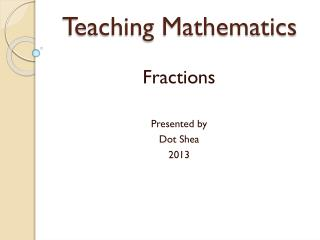DownloadDownload PresentationTeaching Mathematics

# Teaching Mathematics

Télécharger la présentation## Teaching Mathematics

- - - - - - - - - - - - - - - - - - - - - - - - - - - E N D - - - - - - - - - - - - - - - - - - - - - - - - - - -
##### Presentation Transcript

1. Teaching Mathematics Fractions Presented by Dot Shea 2013

2. Fractions • Fractions are about DIVISION • The more you share the less you get • With fractions there are 2 parts to think of: o the PART and o the PART in relation to the WHOLE • Provide different representations / language – linear, area, set

3. Fractions • We come across fractions in many areas of real life • Division- when we share items equally • Money- silver coins • Clocks- ½ past, ¼ to • Measurement: rulers, tape measures, scales, jugs • length-metres • Capacity- litres • Weight- grams • Graphs- representations – 1 bar, picture = 5 • Maps- Scale

4. Area Model Ten frames, Hundred frames Numbers to 10, 100, 1000, fractions, decimals, percentages etc

5. Area Model Fractions • Fractions are not about symmetry. Half is dividing something into two equal parts – Equal area.

6. Fractions Area model

7. Linear Model Number Track: • Knowing how the numbers are related to each other (what comes after this number: how many more to five? How many to 10?) is essential for mental computation, addition and subtraction • The linear model develops proportional understanding through the use of equal spacing and partitioning. This is pre-requisite to number line work.

8. Fractions-Linear Model

9. Set Model A set model is when there are groups of objects

10. Fractions- set model

11. Fractions • To gain a good understanding of fractions students need to be able to read, and manipulate fractions in area, linear and set models.

12. NAPLAN • In the year 5 test 2012, 8 of the questions were directly related to understanding fractions • 11- Length 16-money • 18- Money 20- area • 27- area 28- Time • 35- division 37- division